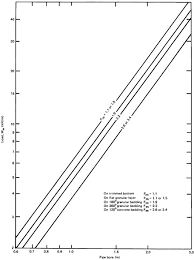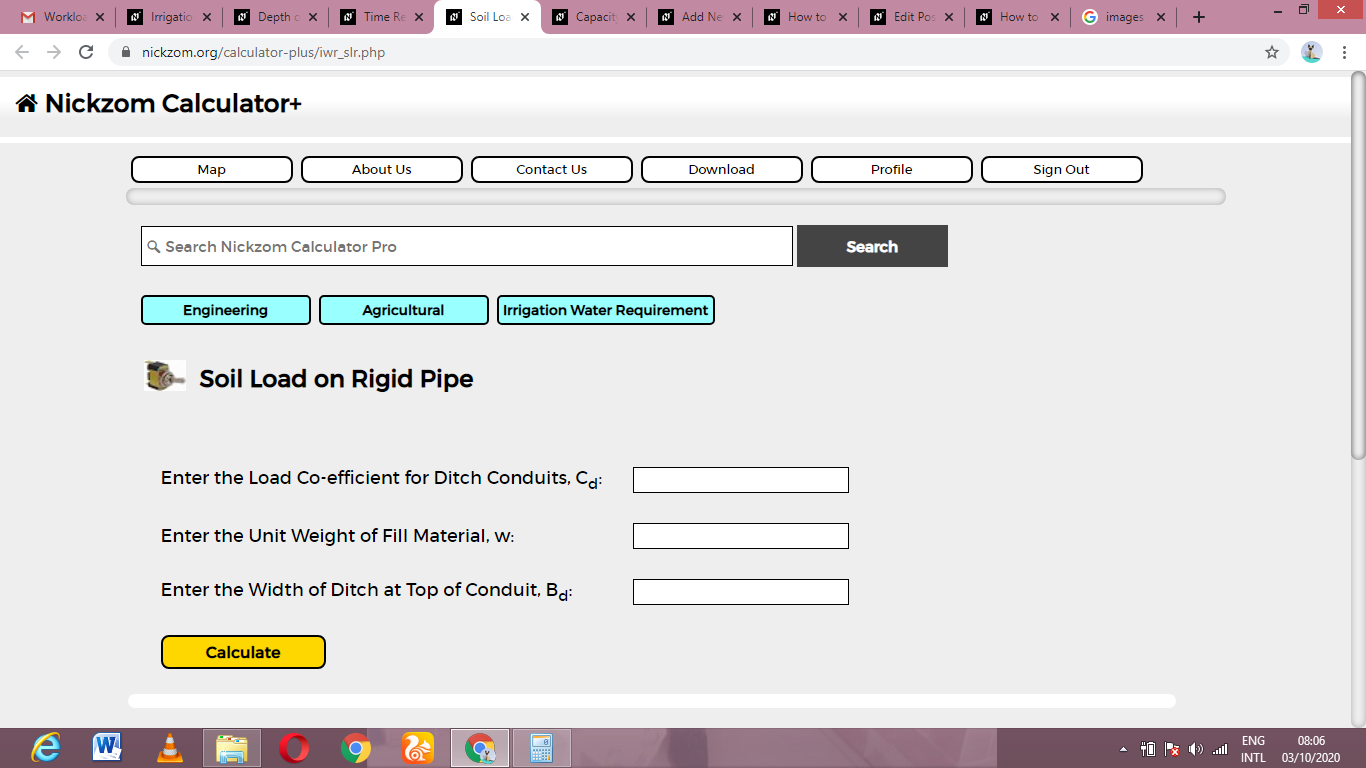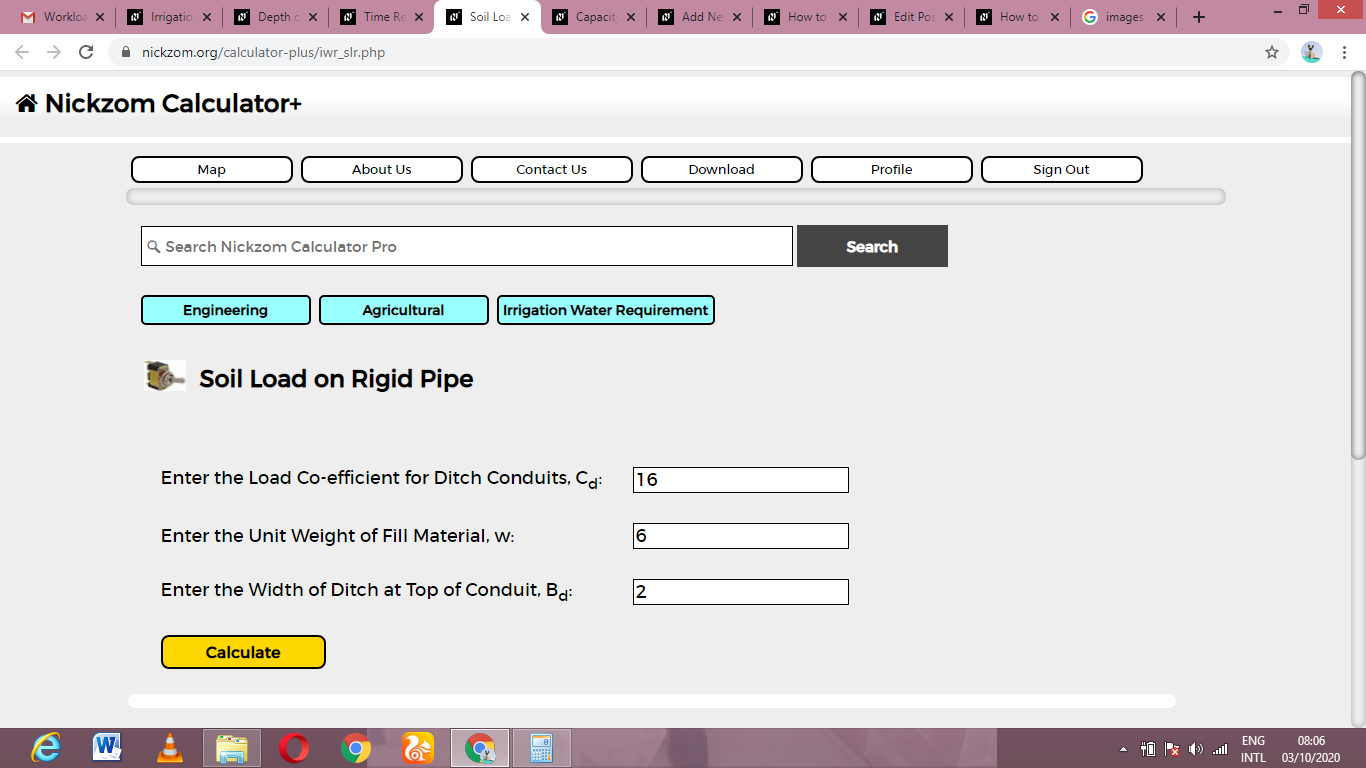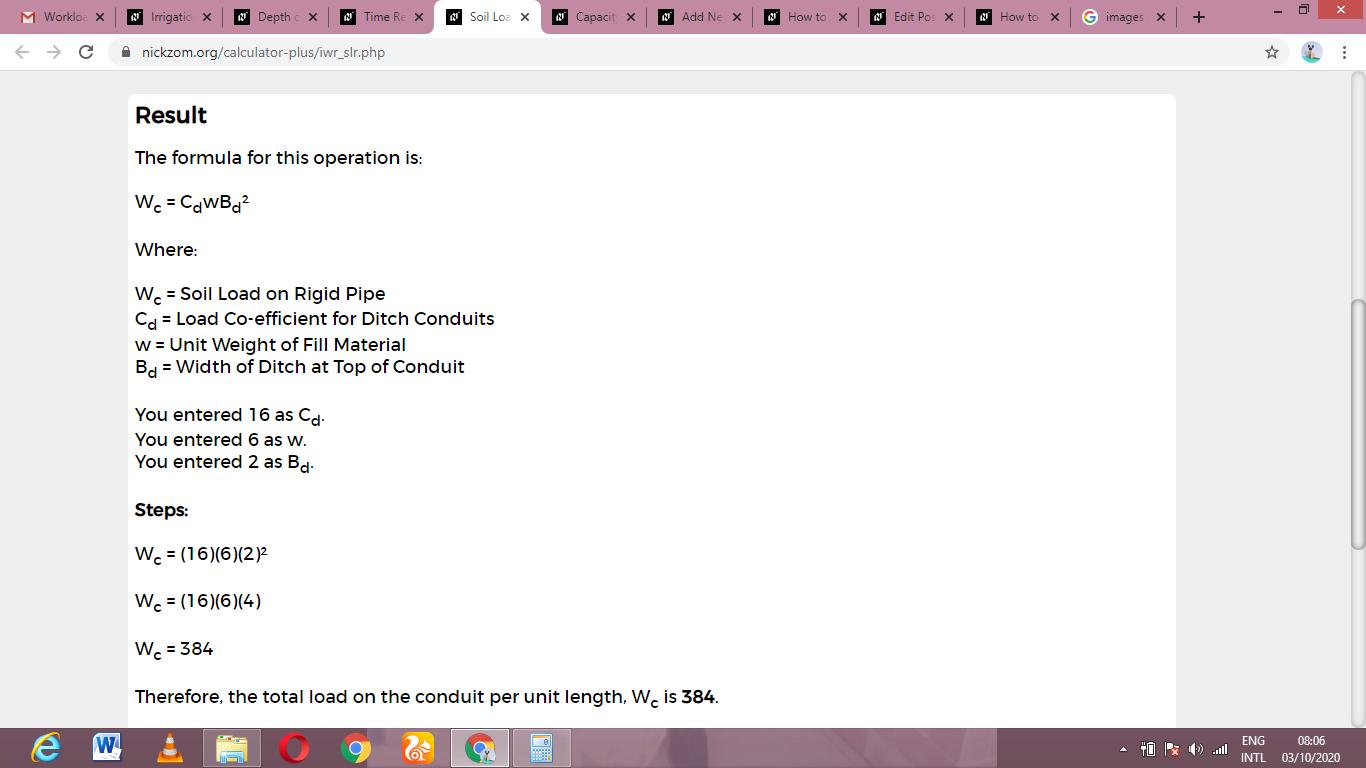# How to Calculate and Solve for Soil Load on Rigid Pipe | Irrigation Water RequirementThe image above represents soil load on rigid pipe.

To compute for soil load on rigid pipe, three essential parameters are needed and these parameters are Load Co-efficient for Ditch Conduits (Cd), Unit Weight of Fill Material (w) and Width of Ditch at Top of Conduit (Bd).

The formula for calculating Soil Load on Rigid Pipe:

Wc = CdwBd²

Where:

Wc = Soil Load on Rigid Pipe
Cd = Load Co-efficient for Ditch Conduits
w = Unit Weight of Fill Material
Bd = Width of Ditch at Top of Conduit

Let’s Solve an example;
Find the soil load on rigid pipe when the load co-efficient for ditch conduits is 16, the unit weight of fill material is 6 and width of ditch at top of conduit is 2.

This implies that;

Cd = Load Co-efficient for Ditch Conduits = 16
w = Unit Weight of Fill Material = 6
Bd = Width of Ditch at Top of Conduit = 2

Wc = CdwBd²
Wc = (16)(6)(2)²
Wc = (16)(6)(4)
Wc = 384

Therefore, the soil load on rigid pipe is 384.

Calculating for the Load Co-efficient for Ditch Conduits when the Soil Load on Rigid Pipe, the Unit Weight of Fill Material and the Width of Ditch at Top of Conduit is Given.

Cd = Wc / w x Bd2

Where;

Cd = Load Co-efficient for Ditch Conduits
Wc = Soil Load on Rigid Pipe
w = Unit Weight of Fill Material
Bd = Width of Ditch at Top of Conduit

Let’s Solve an example;
Find the load co-efficient for ditch conduits when the soil load on rigid pipe is 40, the unit weight of fill material is 4 and the Width of ditch at top of conduit is 2.

This implies that;

Wc = Soil Load on Rigid Pipe = 40
w = Unit Weight of Fill Material = 4
Bd = Width of Ditch at Top of Conduit = 2

Cd = Wc / w x Bd2
Cd = 40 / 4 x 22
Cd = 40 / 4 x 4
Cd = 40 / 16
Cd = 2.5

Therefore, the load co-efficient for ditch conduits is 2.5.

Calculating for the Unit Weight of Fill Material when the Soil Load on Rigid Pipe, the Load Co-efficient for Ditch Conduits and the Width of Ditch at Top of Conduit is Given.

w = Wc / Cd x Bd2

Where;

w = Unit Weight of Fill Material
Wc = Soil Load on Rigid Pipe
Cd = Load Co-efficient for Ditch Conduits
Bd = Width of Ditch at Top of Conduit

Let’s Solve an example;
Find the unit weight of fill material when the soil load on rigid pipe is 62, the load co-efficient for ditch conduits is 2 and the width of ditch at top of conduit is 4.

This implies that;

Wc = Soil Load on Rigid Pipe = 62
Cd = Load Co-efficient for Ditch Conduits = 2
Bd = Width of Ditch at Top of Conduit = 4

w = Wc / Cd x Bd2
w = 62 / 2 x 42
w = 62 / 2 x 16
w = 62 / 32
w = 1.93

Therefore, the unit weight of fill material is 1.93.

Calculating for the Width of Ditch at Top of Conduit when the Soil Load on Rigid Pipe, Load Co-efficient for Ditch Conduits and the Unit Weight of Fill Material is Given.

Bd = √(Wc / Cd x w)

Where;

Bd = Width of Ditch at Top of Conduit
Wc = Soil Load on Rigid Pipe
Cd = Load Co-efficient for Ditch Conduits
w = Unit Weight of Fill Material

Let’s Solve an example;
Find the width of ditch at top of conduit when the soil load on rigid pipe is 72, the load co-efficient for ditch conduits is 3 and the unit weight of fill material is 4.

This implies that;

Wc = Soil Load on Rigid Pipe = 72
Cd = Load Co-efficient for Ditch Conduits = 3
w = Unit Weight of Fill Material = 4

Bd = √(Wc / Cd x w)
Bd = √(72 / 3 x 4)
Bd = √(72 / 12)
Bd = √(6)
Bd = 2.449

Therefore, the width of ditch at top of conduit is 2.45.

Nickzom Calculator – The Calculator Encyclopedia is capable of calculating the soil load on rigid pipe.

To get the answer and workings of the soil load on rigid pipe using the Nickzom Calculator – The Calculator Encyclopedia. First, you need to obtain the app.

You can get this app via any of these means:

To get access to the professional version via web, you need to register and subscribe for NGN 2,000 per annum to have utter access to all functionalities.
You can also try the demo version via https://www.nickzom.org/calculator

Apple (Paid) – https://itunes.apple.com/us/app/nickzom-calculator/id1331162702?mt=8
Once, you have obtained the calculator encyclopedia app, proceed to the Calculator Map, then click on Agricultural under Engineering.Now, Click on Irrigation Water Requirement under AgriculturalNow, Click on Soil Load on Rigid Pipe under Irrigation Water RequirementThe screenshot below displays the page or activity to enter your values, to get the answer for the soil load on rigid pipe according to the respective parameters which is the Load Co-efficient for Ditch Conduits (Cd), Unit Weight of Fill Material (w) and Width of Ditch at Top of Conduit (Bd).Now, enter the values appropriately and accordingly for the parameters as required by the Load Co-efficient for Ditch Conduits (Cd) is 16, Unit Weight of Fill Material (w) is 6 and Width of Ditch at Top of Conduit (Bd) is 2.Finally, Click on CalculateAs you can see from the screenshot above, Nickzom Calculator– The Calculator Encyclopedia solves for the soil load on rigid pipe and presents the formula, workings and steps too.